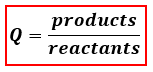# Problem: For the reaction:PCl3 (g) + Cl2 (g) ⇌ PCl5 (g)           K c = 24.3a system is prepared with[PCl3] = 0.10 M; [Cl 2] = 0.15 M; [PCl 5] = 0.60 M.Which response is correct? a. the reaction is at equilibrium and concentrations will not changeb. the amount of PCl 5 will increasec. more PCl3 will formd. the reaction will shift righte. the amount of PCl 3 will increase while the concentration of Cl 2 will decrease.

###### FREE Expert Solution
84% (382 ratings)
###### FREE Expert Solution

We are being asked what would be the reaction condition of the given reaction mixture given the concentrations of the reactants and product. We will use the reaction quotient, Q, to determine if a chemical reaction would be at equilibrium or not. The formula for Q is:Depending on if Q is greater than or less than K our reaction will shift to attain equilibrium by reaching the equilibrium constant K:

84% (382 ratings)###### Problem Details

For the reaction:

PCl3 (g) + Cl2 (g) ⇌ PCl5 (g)           K c = 24.3

a system is prepared with

[PCl3] = 0.10 M; [Cl 2] = 0.15 M; [PCl 5] = 0.60 M.

Which response is correct?

a. the reaction is at equilibrium and concentrations will not change

b. the amount of PCl 5 will increase

c. more PCl3 will form

d. the reaction will shift right

e. the amount of PCl 3 will increase while the concentration of Cl 2 will decrease.